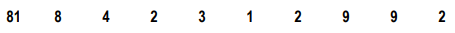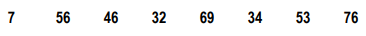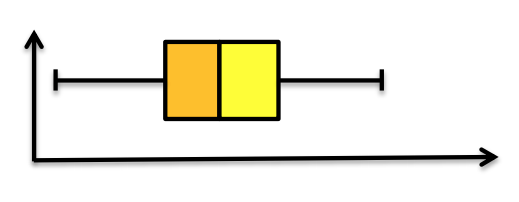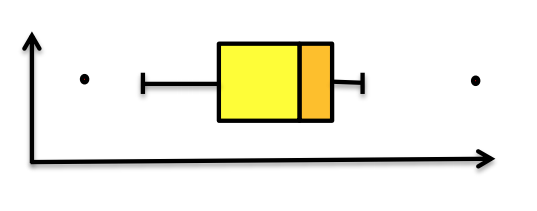Ch.2: Measures of Center and SpreadWorksheetSee all chapters
 Ch.1: Displaying Numeric Data 1hr & 19mins 0% complete Worksheet Ch.2: Measures of Center and Spread 2hrs & 18mins 0% complete Worksheet Ch.3: Probability and Rules 1hr & 44mins 0% complete Worksheet Ch.4: The Discrete Random Variable 53mins 0% complete Worksheet Ch.5: The Binomial Random Variable 1hr & 38mins 0% complete Worksheet Ch.6: Types of Continuous Random Variable Distributions 1hr & 35mins 0% complete Worksheet Ch.7: The Standard Normal Distribution (Z-Scores) 1hr & 22mins 0% complete Worksheet Ch.8: Using The Z-Score 1hr & 24mins 0% complete Worksheet Ch.9: Sampling Distributions: Mean 1hr & 22mins 0% complete Worksheet Ch.10: Sampling Distributions: Proportion 1hr & 31mins 0% complete Worksheet Ch.11: Hypothesis Testing: Part 1 1hr & 42mins 0% complete Worksheet Ch.12: Hypothesis Testing: Part 2 1hr & 43mins 0% complete Worksheet

# Boxplots (The Five Number Summary)

See all sections

Concept #1: Determining The Five Number Summary and Drawing Boxplots

Concept #2: Determining The Five Number Summary and Drawing Boxplots: Intro

Practice: Determine the mean and median for the following data set:Practice: Determine the mean and median for the following data set:Practice: From the boxplot in Practice 2, what can be said about the data set in Practice 1?

Practice: What can be said about the data set that generated the boxplot below?Practice: What can be said about the data set that generated the boxplot below?